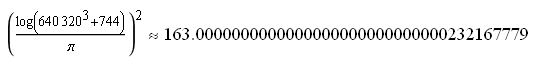# 163

There is a reasonably popular card game entitled 24, where players attempt to derive 24 by applying basic arithmetic operations to a set of four positive integers. For example, the provided integers may be {3, 3, 8, 8}. A solution must use all of the provided integers, so 3*8 is an invalid solution. The actual solution is difficult to find, being 8/(3-(8/3)). This can be represented as a tree:The number of ways in which four integers {a, b, c, d} can interact with four operations {+, *, /, -} is rather large. An upper bound is 7680, which is the number of fully-parenthesised expressions containing the four integers (in some permutation) separated with arithmetic operations. This is the product of the number of permutations (4! = 24), parenthesisations (C(3) = 5) and sequences of operations (4^3 = 64). The actual number of possibilities is considerably smaller, as I have not taken into account the commutativity or associativity of + and *.

I heard that a variant of this game is 163. You begin with a few randomly chosen playing cards (where Jack, Queen and King represent 11, 12 and 13, respectively) and attempt to obtain a value as close as possible to 163. Being the largest Heegner number, there is a very elegant formula approximating 163, discovered by Srinivasa Ramanujan:The logarithm is, of course, to base e.

This entry was posted in Uncategorized. Bookmark the permalink.